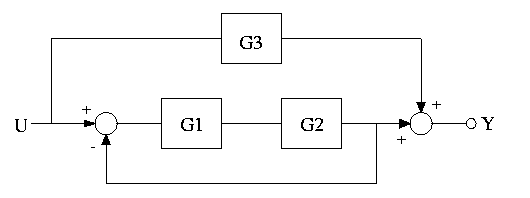Ch Control System Toolkit Demos Example 7 -- Calculating Transfer Function The system show below consists of three subsystems with transfer functions G1 = 1/(s+1), G2 = 1/s, G3 = 2. Find the transfer function of this system.Answer: The transfer function of this system is 2.00*s^2 + 2.00*s + 3.00 G(s) = ------------------------ 1.00*s^2 + 1.00*s + 1.00